# Excel: If cell contains then count, sum, highlight, copy or delete

In our previous tutorial, we were looking at Excel If contains formulas that return some value to another column if a target cell contains a given value. Aside from that, what else can you do if a cell contains specific text or number? A variety of things such as counting or summing cells, highlighting, removing or copying entire rows, and more.

## Excel 'Count if cell contains' formula examples

In Microsoft Excel, there are two functions to count cells based on their values, COUNTIF and COUNTIFS. These functions cover most, though not all, scenarios. The below examples will teach you how to choose an appropriate Count if cell contains formula for your particular task.

### Count if cell contains any text

In situations when you want to count cells containing any text, use the asterisk wildcard character as the criteria in your COUNTIF formula:

COUNTIF(range,"*")

Or, use the SUMPRODUCT function in combination with ISTEXT:

SUMPRODUCT(--(ISTEX(range)))

In the second formula, the ISTEXT function evaluates each cell in the specified range and returns an array of TRUE (text) and FALSE (not text) values; the double unary operator (--) coerces TRUE and FALSE into 1's and 0's; and SUMPRODUCT adds up the numbers.

As shown in the screenshot below, both formulas yield the same result:

`=COUNTIF(A2:A10,"*")`

`=SUMPRODUCT(--(ISTEXT(A2:A10)))`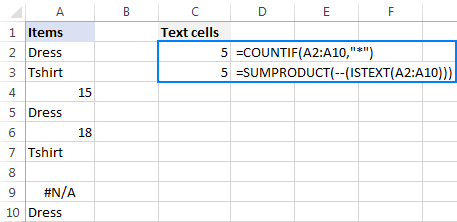### Count if cell contains specific text

To count cells that contain specific text, use a simple COUNTIF formula like shown below, where range is the cells to check and text is the text string to search for or a reference to the cell containing the text string.

COUNTIF(range,"text")

For example, to count cells in the range A2:A10 that contain the word "dress", use this formula:

`=COUNTIF(A2:A10, "dress")`

Or the one shown in the screenshot: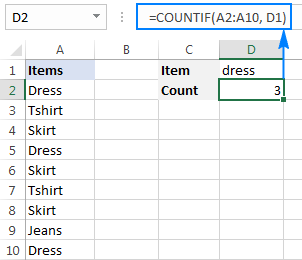### Count if cell contains text (partial match)

To count cells that contain a certain substring, use the COUNTIF function with the asterisk wildcard character (*).

For example, to count how many cells in column A contain "dress" as part of their contents, use this formula:

`=COUNTIF(A2:A10,"*dress*")`

Or, type the desired text in some cell and concatenate that cell with the wildcard characters:

`=COUNTIF(A2:A10,"*"&D1&"*")`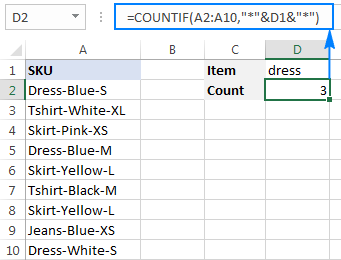### Count if cell contains multiple substrings (AND logic)

To count cells with multiple conditions, use the COUNTIFS function. Excel COUNTIFS can handle up to 127 range/criteria pairs, and only cells that meet all of the specified conditions will be counted.

For example, to find out how many cells in column A contain "dress" AND "blue", use one of the following formulas:

`=COUNTIFS(A2:A10,"*dress*", A2:A10,"*blue*")`

Or

`=COUNTIFS(A2:A10,"*"&D1&"*", A2:A10,"*"&D2&"*")`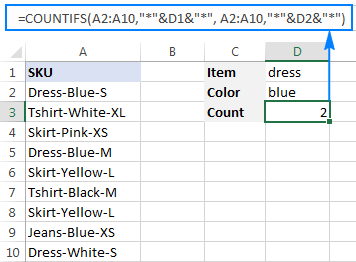### Count if cell contains number

The formula to count cells with numbers is the simplest formula one could imagine:

COUNT(range)

Please keep in mind that the COUNT function in Excel counts cells containing any numeric value including numbers, dates and times, because in terms of Excel the last two are also numbers.

In our case, the formula goes as follows:

`=COUNT(A2:A10)`

To count cells that DO NOT contain numbers, use the SUMPRODUCT function together with ISNUMBER and NOT:

`=SUMPRODUCT(--NOT(ISNUMBER(A2:A10)))`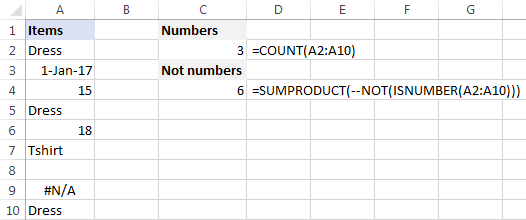## Sum if cell contains text

If you are looking for an Excel formula to find cells containing specific text and sum the corresponding values in another column, use the SUMIF function.

For example, to find out how many dresses are in stock, use this formula:

`=SUMIF(A2:A10,"*dress*",B2:B10)`

Where A2:A10 are the text values to check and B2:B10 are the numbers to sum.

Or, put the substring of interest in some cell (E1), and reference that cell in your formula, as shown in the screenshot below: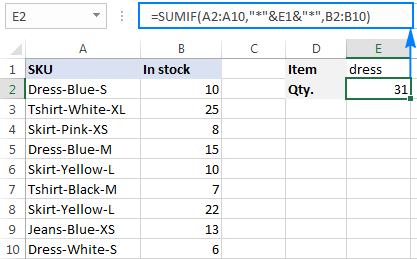To sum with multiple criteria, use the SUMIFS function.

For instance, to find out how many blue dresses are available, go with this formula:

`=SUMIFS(B2:B10, A2:A10,"*dress*",A2:A10,"*blue*")`

Or use this one:

`=SUMIFS(B2:B10, A2:A10,"*"&E1&"*",A2:A10,"*"&E2&"*")`

Where A2:A10 are the cells to check and B2:B10 are the cells to sum.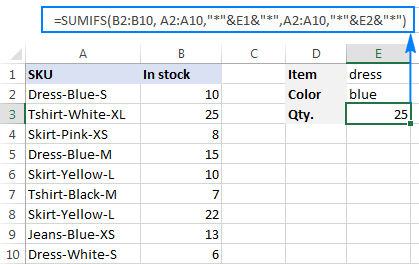## Perform different calculations based on cell value

In our last tutorial, we discussed three different formulas to test multiple conditions and return different values depending on the results of those tests. And now, let's see how you can perform different calculations depending on the value in a target cell.

Supposing you have sales numbers in column B and want to calculate bonuses based on those numbers: if a sale is over \$300, the bonus is 10%; for sales between \$201 and \$300 the bonus is 7%; for sales between \$101 and \$200 the bonus is 5%, and no bonus for under \$100 sales.

To have it done, simply multiply the sales (B2) by a corresponding percentage. How do you know which percentage to multiply by? By testing different conditions with nested IFs:

`=B2*IF(B2>=300,10%, IF(B2>=200,7%, IF(B2>=100,5%,0)))`

In real-life worksheets, it may be more convenient to input percentages in separate cells and reference those cells in your formula:

`=B2*IF(B2>=300,\$F\$5,IF(B2>=200,\$F\$4,IF(B2>=100,\$F\$3,\$F\$2)))`

The key thing is fixing the bonus cells' references with the \$ sign to prevent them from changing when you copy the formula down the column.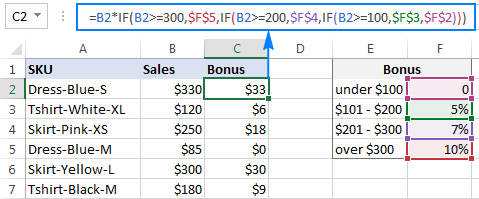## Excel conditional formatting if cell contains specific text

If you want to highlight cells with certain text, set up an Excel conditional formatting rule based on one of the following formulas.

### Case-insensitive:

SEARCH("text", topmost_cell)>0

### Case-sensitive:

FIND("text", topmost_cell)>0

For example, to highlight SKUs that contain the words "dress", make a conditional formatting rule with the below formula and apply it to as many cells in column A as you need beginning with cell A2:

`=SEARCH("dress", A2)>0`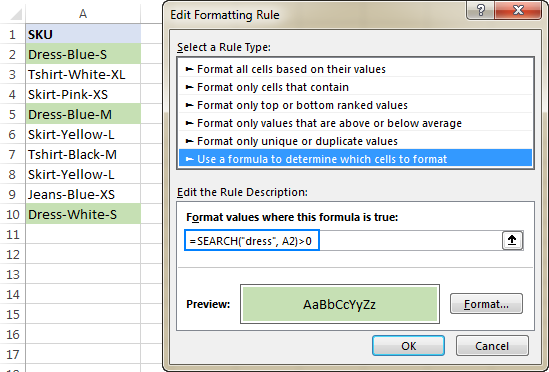### Excel conditional formatting formula: if cell contains text (multiple conditions)

To highlight cells that contain two or more text strings, nest several Search functions within an AND formula. For example, to highlight "blue dress" cells, create a rule based on this formula:

`=AND(SEARCH("dress", A2)>0, SEARCH("blue", A2)>0)`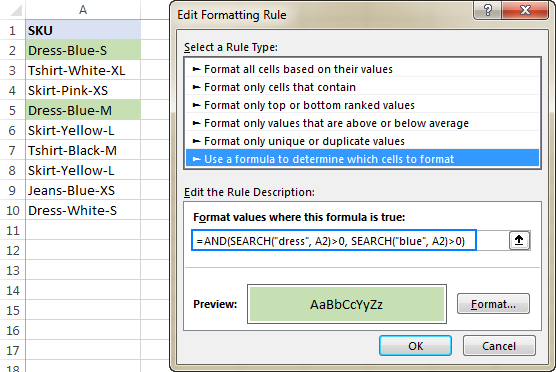For the detailed steps, please see How to create a conditional formatting rule with a formula.

## If cell contains certain text, remove entire row

In case you want to delete rows containing specific text, use Excel's Find and Replace feature in this way:

1. Select all cells you want to check.
2. Press Ctrl + F to open the Find and Replace dialog box.
3. In the Find what box, type the text or number you are looking for, and click the Find All
4. Click on any search result, and then press Ctrl + A to select all.
5. Click the Close button to close the Find and Replace
6. Press Ctrl and the minus button at the same time (Ctrl -), which is the Excel shortcut for Delete.
7. In the Delete dialog box, select Entire row, and click OK. Done!

In the screenshot below, we are deleting rows containing "dress":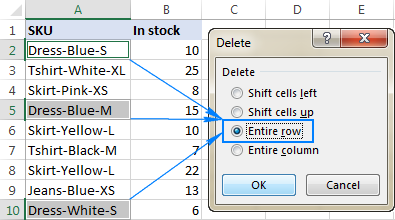## If cell contains, select or copy entire rows

In situations when you want to select or copy rows with relevant data, use Excel's AutoFilter to filter such rows. After that, press Ctrl + A to select the filtered data, Ctrl+C to copy it, and Ctrl+V to paste the data to another location.

To filter cells with two or more criteria, use Advanced Filter to find such cells, and then copy the entire rows with the results or extract only specific columns.

For the detailed step-by-step instructions, please see the following tutorials:

This is how you manipulate cells based on their value in Excel. To have a closer look at the formulas discussed in this tutorial, you are welcome to download our sample Excel If Cell Contains workbook. I thank you for reading and hope to see you on our blog next week!

## You may also be interested in

### 84 comments to "Excel: If cell contains then count, sum, highlight, copy or delete"

1.Valerie says:

Hello,

I would appreciate your help on a formula.

I am creating a vertical employee absence calendar in Excel (dates at the top, names on the side) with leave entered as text: holiday (H), half day holiday (HH), sick leave (S), and half day sick leave (SH).

I would like to add a row total for each person.

With COUNTA all entries = 1
So for e.g. H + S + HH should add up to 2.5 but COUNTA shows 3.

How can Excel add up different values for each type of text? So that that H = 1, HH = 0.5, S=1 and SH=0.5?

Valerie

•Alexander Trifuntov (Ablebits Team) says:

Hi!
The COUNTA function counts the number of cells. So it cannot return a fractional number. Replace letters with numbers using the IF function:

=IF(A1="H",1,0)+IF(A2="HH",0.5,0)

2.Mustafeez says:

Hi

how I sum if I had 3 table
1 vendor (Samsung or Huawei)
2 Cell mode (ex Samsung I9500 series)
3 count

I used below formula but it count other vendor model as well

=SUMIF(J2:J23068,"*I9500*",K2:K23068)

can you please support for the correction

•Alexander Trifuntov (Ablebits Team) says:

Hi!
To calculate the sum of two criteria, use the SUMIFS function.

•Mahaveer Arvind says:

Hi
I need your support for correction of formula.

•Mahaveer Arvind says:

Please check below this formula is correct or not

=SUM((Sheet2!\$D\$8:\$QC\$11)*(--(Sheet2!\$C\$8:\$C\$11='Cleaning details'!\$D6))*(--(Sheet2!\$D\$4:\$QC\$4='Cleaning details'!\$C6))*(--(Sheet2!\$D\$3:\$QC\$3='Cleaning details'!\$E\$4)))+SUM(Sheet2!\$D\$12:\$QC\$15)*(--(Sheet2!\$C\$12:\$C\$15='Cleaning details'!\$D6))*(--(Sheet2!\$D\$5:\$QC\$5='Cleaning details'!\$C6))*(--(Sheet2!\$D\$3:\$QC\$3='Cleaning details'!\$E\$4))+SUM(Sheet2!\$D\$16:\$QC\$19)*(--(Sheet2!\$C\$16:\$C\$19='Cleaning details'!\$D6))*(--(Sheet2!\$D\$6:\$QC\$6='Cleaning details'!\$C6))*(--(Sheet2!\$D\$3:\$QC\$3='Cleaning details'!\$E\$4))+SUM(Sheet2!\$D\$20:\$QC\$23)*(--(Sheet2!\$C\$20:\$C\$23='Cleaning details'!\$D6))*(--(Sheet2!\$D\$7:\$QC\$7='Cleaning details'!\$C6))*(--(Sheet2!\$D\$3:\$QC\$3='Cleaning details'!\$E\$4))

•Mahaveer Arvind says:

Because this is not working in single cell.

•Alexander Trifuntov (Ablebits Team) says:

Hi!
It is very difficult to understand a formula that contains unique references to your workbook worksheets. Hence, I cannot check its work, sorry.

3.Rob says:

Screenshot link to reference my sheet: https://gyazo.com/36d77c6c536984cfe9b56d1bc51b6e09

Hi, my business gives commissions to our drivers (called “captains” as shown in the Excel screenshot). Their commission is 10% of the sale price of a surcharge they sell.

I want to track their daily commissions per captain and then total each daily commission for their weekly total commission per captain.

I already have their daily tracking set up to automatically calculate their daily commissions based on the prices of surcharges and tally of quantity sold for each.

My issue now is making the formula that automatically searches row P5 to T5 for instances of their unique name being written. Each time their name is written in that row indicates one working day with commissions made (total for each daily commission is in row P30 to T30) in their own column.

I would then like the same formula to have N34 to N36 autofill if there are captain’s names found in P5 to T5 (without duplicating their names) and then totaling their daily commissions specific to each captain’s name in the weekly totals at O34 to O36.

I hope this makes sense. Let me know if you need clarification.

I’ve tried for several hours to figure it out with various formulas but I’m not too versed in Excel so I’m hoping someone can help me out. Thanks in advance!

•Alexander Trifuntov (Ablebits Team) says:

Hi!

Sorry, there is no simple solution for your task. At least I do not know such :(

4.Jyothi Prasad says:

Hi,
SUMIF function is working for me but SUMIFS is not working even though I tried so many times. =SUMIFS(J2:J29,G2:G29,"Passive",G2:G29,"Connector") is the formula which I am trying in my excel. I also have used * prior to text values but no result and instead its showing 0. I can't understand why its showing 0. Kindly help.

•Alexander Trifuntov (Ablebits Team) says:

Hello!
I can't see your data and can't check, but this formula should work

=SUMIFS(J2:J29,G2:G29,”*Passive*”) + SUMIFS(J2:J29,G2:G29,”*Connector*”)

I recommend reading this guide: Excel SUMIF with multiple criteria (OR logic).
Hope this is what you need.

5.Debbie says:

I have an Excel sheet where in column A i have various dates for sales over a three year period and in column B I have another column which contains text relating to the type of sale, either "Direct" or "Indirect" to indicate if the sale was a direct sale or through another party. I want to be able to count how many Direct or Indirect sales were made in each year. Can you help? I have tried combining the COUNTIF and COUNTIFS formulas but it's not working. I can get the number of sales in each year by using the COUNTIFS formula but the problem starts when I try to extract the count in each year for each of Direct or Indirect by combining the two formulas. Thanks! Your website is amazing! I've managed to solve a lot of problems by checking your solutions.

•Alexander Trifuntov (Ablebits Team) says:

Hello!
What formulas are you using? What's not working in them? You must use the COUNTIFS formula with two conditions - Date and Sales Type.
If they don’t work for you, then please describe your task in detail, I’ll try to suggest a solution.

6.Natalia says:

Hello,

What formula could I use to add up both numbers and letters in the same column? For example, I have 12 cells with values in the same column (the "x" respresents 1):

10
x
x
2
3
x
x
x
x
x
x
x

Before the numbers were added and we were only counting "x", we used this formula =COUNTIF(G73:G85,"=x")

Now that numbers are also included, I am having trouble finding a formula that adds both "x" as 1 and the rest of the numbers.

Thank you!

•Alexander Trifuntov (Ablebits Team) says:

Hello!
If I understand your task correctly, the following formula should work for you:

=SUM(A1:A10)+COUNTIF(A1:A10,"x")

SUM function only adds numbers and ignores text.

7.Anna says:

Hi,

I have two tabs:

First tab:
Column A Name
Column B Yes ( or No)

For example :
Column A Column B
Anna Yes
Lily No

If column B is value is Yes, I want to add column A value (Anna) to the other tab A1.
If column B is value is No, I don't want to add column A value (Lily) into the other tab.

How to achieve that?

Thanks,

8.KALEB says:

I am having a really hard time trying to get the formula I need. The spreadsheet is for stock options. For easy example, column A are debits that it cost to open the contract. The adjacent cell in column B is the price I sell to close the contract. I have everything I need for the gain/loss and percentage taken. I am trying to figure out how I would calculate my true return? if for example I open a contract for -\$500 and sell it for \$750, I have a return of 50% on that specific row. However if I open another trade using that same \$500 and then the \$250 I captured for a trade as well, how would I calculate my overall return? It would want to calculate my return on \$1250 instead of \$750. Any help would be greatly appreciated.

•Alexander Trifuntov (Ablebits Team) says:

Hi!
We are not stock specialists. If you have a specific question about the Excel formula, we will try to help.

9.renzel says:

Trying to make a formula using sumifs in B1 "lol" && C1 "wow

A1 blank cell (i want to put name text here which will be the reference of A2:A10)
B1 show the total lol
C1 show the total wow
A2:A10 Names
B2:B10 [sum_range]
C2:C10 text "Lol" or "wow"

I want to sum the value in B2:B10 if it meets the condition:
Sumif:
Condition 1 If A2:A10 is true (or same with the text I input in A1)
Condition 2 If C2:C10 "lol" or in "wow" category

e.q.
A1 "10/06"
B1 (if A1 is true A2;A10 sum the total 10/06 with lol
C1 sum the total 10/06 wow

•Alexander Trifuntov (Ablebits Team) says:

Hello!
To find the sum of values for conditions, use the SUMIFS function.

10.Ravin Kayasth says:

Hey Alex,
At the outset, very wonderfull site and informative.
I have the data in the following cols
Col A Col B
21-Sep-21 55
23-Sep-21 74
02-Oct-21 21
05-Oct-21 05

All I want to do is add the values apprearing for Sep and Oct as below
Sep'21 xxxxx
Oct'21 yyy

•Alexander Trifuntov (Ablebits Team) says:

Hi!
I don't understand what you want to do. Explain in more detail.

11.Rere says:

I am trying to find a formula to calculate cum GPA but will subtract the grades that have "TR" by it in the semester column. What formula if any would work for this?
EXAMPLE

A 3 12 SP20
B 3 9 TR
C 2 4 FA21
D 1 1 TR

12.anum says:

I need help with this formula. =SUMIF(\$D:\$D,"*Wave 1*",\$F:\$F)
Right now I want to add values from F if D says Wave 1 however if the the sum is 0 i want it to say TBD .. not sure how to do the TBD part

•Alexander Trifuntov (Ablebits Team) says:

Hi!

13.Thara says:

Hi,
I need to add values in alternative cells. eg: values in I5,K5,M5 and the sum should ignore text in those cells. Please suggest

14.Mohanakrishnan M says:

In fact i would like to know whether excel can display the value of a cell in another cell by using an IF condition.
e.g value of A1 is "FLOWERS"
input text "FL" in B1
the result should be the value of A1 to be displayed in C1 if you put a condition that if the value of B1 = "FL"
is there any formula to solve this.

thanks

•Alexander Trifuntov (Ablebits Team) says:

Hello!

IF(B1="FL",A1,"")

15.Celeste says:

I have a list of parts in column A, then i have a list of dates in column B
I want the formula to return how many times each part number has a date beside it
123456 1-jan-21
234567 5-jan-21
123456
123456 5-feb-21

so i would want this to return
123456 2
234567 1

•Alexander Trifuntov (Ablebits Team) says:

Hello!

=COUNTIFS(A1:A100,D1,B1:B100," < > "&"")

D1="123456"

16.Madeleine C says:

Please help me figure this out. I have a spreadsheet of pupils' scores on a test. I have put a '1' in a cell if they got the question correct, a '0' if incorrect and a '.' if they didn't attempt it. I have a separate list to the side which says the name of the topic next to each question number. I would like to generate a list of topics they need to practice for each pupil based on the questions they got wrong. How can I do this? For example, if they got question 8 and 10 incorrect and these questions were a multiplication and a division question I would like a list which says multiplication and division. Please help me figure this out!

•Alexander Trifuntov (Ablebits Team) says:

Hello!
Please use the following array formula -

{=IFERROR(INDEX(\$C\$3:\$C\$30, SMALL(IF(1=((--(\$I3=\$A\$3:\$A\$30))*(--(\$J3=\$B\$3:\$B\$30))), ROW(\$C\$3:\$C\$30)-2,""), COLUMN()-10)),"")}

A3:C30 - your table, I3 - name, J3 - zero or "." K3 - this formula.
Please have a look at this article - Vlookup multiple matches and return results in a row (Formula 2)

17.CRX says:

Hi Everyone, I would really like some help with this logic

I would like to create a formula in cell C2, if D2 = text "X" perform sum of A2 + B2, but if D2 = text "W" cell = just the value in B2

I hope this makes sense, TIA

18.Moin says:

If all my cells contains value,(without blank) then I should get the output as T else F

19.John says:

What I'm looking for is a formula that will do the following:
If cell a1 contains specific text, then count cell a2.

I've tried all sorts of different options, but can't get it to show the right count.

•Alexander Trifuntov (Ablebits Team) says:

Hello!
You can check the text written in the cell using the ISTEXT function. Use it in an IF function statement. I don't quite understand what "count cell a2" means.

•John says:

Here is an example of what I've attempting. Perhaps this might help.

If in the range of data, X appears, then count the next cell after X (which would have A, B, or C in it).

20.Cherine says:

Thank you for all the Information they are great. what i would like to dois:
I Have in column A a data validation that has X,Y Z and on a different sheet under X i have a list of names in a column as well as under Y ans Z. what I want, is when I choose for example X in column A, I want in column B automatically to copy all the names of the other sheet under X into the cells in column B into a column not row? please can you help me with this problem?

21.Ludmila Neville says:

Hello, could you please help me to find formula that highlights the cell with specific text when the text is a list? Is this the correct way to do it in Conditional Formatting (by formula)?
=ISNUMBER(MATCH(\$A\$2,List,0))
when list is the range of list cells?
If this is the best way, Is there a way to copy this exact formatting to other cells (format painter doesn't do the job)?
Thank you so much in advance.

•Alexander Trifuntov (Ablebits Team) says:

Hello Ludmila!
If I understand your task correctly, the following conditional formatting formula should work for you:

=ISNUMBER(MATCH(\$A\$2,F2,0))

where F2 is the first cell of the list cell range.

•Ludmila Neville says:

Hello Alexander, thank you for your answer. Unfortunately, the formula you've suggested didn't work for me, though the formula that includes range of cells containing the list works well.
My additional question was is there a way to apply the same conditional formatting formula to multiple cells. I mean is there a fast way to copy the conditional formatting formula that will be updated to other cells (eg A3, A4 and so on) instead of creating a new rule for every next cell? Thank you!

•Alexander Trifuntov (Ablebits Team) says:

Hello!
I'm sorry the formula wasn't useful to you. Apparently, we represent your data differently.
How to copy conditional formatting is described here.

22.Ebadullah says:

I am looking to use a condition on one cell (A2=Kabul, then pick data from another sheet, K5) and the same condition will be repeated in one formula (A2=Laghman, A2=Logar..... and then data will be picked from a different cell). How I can do it?

•Alexander Trifuntov (Ablebits Team) says:

For me to be able to help you better, please describe your task in more detail. Please let me know in more detail what you were trying to find, what formula you used and what problem or error occurred. Give an example of the source data and the expected result. It’ll help me understand it better and find a solution for you. Thank you.

23.Simon says:

Hi there,
How do I Sum out multiple SKUs when I keyed them in according to the warehouse racking layout?
Example :
A1 B1 C1 are the name of the SKUs, while A1 C1 has the same SKU.
A2 B2 C2 will be the Quantity of each SKU above.
How do I get the the sum of A2 and C2 in a separate list if we got 600 SKUs.
Thank you.

24.Gbenga says:

Please if cell b4 has a data of 24pcs and cell c4 has 34000....
I want to multiply the two cell together...
Thanks

•Alexander Trifuntov (Ablebits Team) says:

Hello Gbenga!
If I understand your task correctly, the following formula should work for you

=LEFT(A1,LEN(A1) - FIND("pcs",A1)) * B1

Hope you’ll find this information helpful.

25.Alexander Trifuntov (Ablebits Team) says:

Hello George!
In order to extract all the characters after "UL9", use the formula below:
=RIGHT(A10, LEN(A1) - FIND("UL9", A1,1) +1)

As for pulling the text before "UL9", here is the formula for this case:
=LEFT(A1, FIND("UL9", A1 ,1) - 1)

BTW, there is a formula-free solution for this task called Extract Text tool. Check out its manual, I believe you'll find it helpful: https://www.ablebits.com/docs/excel-extract-text/

26.george papaliberios says:

How can I delete upon scanning a bar code, everything except the text that starts with UL9C0037365 value in a cell
01008568230065341119071521UL9C0037365

Thank you
george

27.Harry says:

Thank you so much for most helpful forumula

28.Eddie says:

How do I have a cell calculate a sum if there's a certain word or text in another cell? I.E. 12345*2% will only be calculated if say cell A5 was showing the word "No".
Thanks!

29.Scott says:

I need a formula that will search a specific cell (E2) or all of column E for a "key" word and then if found, take the date in A2 and add 30 days to it and put this all in B2.

Please let me know if this can be accomplished.
Thanks.

•Nicola Lovell says:

Hello,

Did you find a solution for your issue? I am currently trying to find something similar. I need to find all people who are online and add their hours, ignoring those who are on leave etc, Can anyone help?

30.Dinesh Kommireddy says:

Hi,
Is there a solution for this?

Basically, I have month in one column and dollars in another. If I want the sum of all the dollars in June, July and Aaugust in a separate column. Is there a formula that does this. I am sure there is but cannot figure it out.

Thanks,
Dinesh.

Month dollar sum
June 20
June 30
June 25
June 22
June 31 sum of June dollars
July 18
July 16
July 15
July 22
July 19 sum of July dollars
August 6
August 3
August 32
August 34
August 24 sum of Aug dollars

31.Eric Moore says:

Any Help Apprecaiated:
Essentially I am trying to write a formula where if all cells in a column range contain a numerical value it will SUM them, but add a condition where if the column contains a text it will search for the text and instead of returning a SUM it will return "Not Complete".

Currently I am working with the following formula but cant seem to get it to work:
=IF(ISNUMBER(SEARCH("Not Complete",H4:\$H2000)),"Not Complete",SUM(H4:\$H2000))

For Reference:
Column of values mixed with text containing "Not Complete"
\$0.052000
\$0.052000
\$0.052000
\$0.052000
Not Complete
Not Complete
Not Complete
Not Complete
\$0.052000
\$0.052000
\$0.052000

32.Simon Hunt says:

Trying to make a formula where if a cell has the text "GMP 1" then show the value which is in the same row but from column A. Also this has to work over separate sheets.
So, for example, i need it to look through column C on sheet 1 for the word "GMP 1" but return the row value of Column A, and put the result on sheet 2. Currently i have multiple efforts at the formula and getting results where it adds up the values from A (which i dont want) or #value #n/a or just the count value of if it is true. Tried multiple different starting points (sumproducts/vlookup etc)

•Roz says:

Did anyone ever answer you? I'm looking for the same solution and can't figure it out for the life of me. Thanks!

33.Sri says:

C5=Q
D5=Q
E5=H
F5=H
G5=Q
H5=Q
I5=H
J5=Q
K5=H
L5=H
M5=H
N5=H
O5=Q
P5=Q
Q is the travel plan for onsite. for the above example its 7 weeks stays.
but the onsite trip would be 4. so i need to caluclate the trip count.
I want to have a successive cell count where the value is "Q"
For the above example it would be 4.
Thanks
Srini

34.nick c says:

I am trying to see if excel can search a column and automatically add up specific cells to come as close to without exceeding a specific number. Example: I have a 12' (120") piece of lumber and I need to cut that into as many posts as possible with little waste. So in the example below it would highlight cells (A1, A2, A5, A6) in a specific color, then in another color continue with the other cells (not previously selected) and perform the same function. Thanks for any help.
A1 13.5"
A2 25.75"
A3 54"
A4 32"
A5 16"
A6 64.75"
A7 10"
A8 12.5"
A9 11

35.David Lee says:

I'm working on a spreadsheet where i would like for a formula to be created when any text is entered into the cell to the left. If you enter a company name in a cell it will then create a PO based off the job number (in one cell) & the cost code (another cell)
Cell B2 is My Job Number & Cell A14 is My Cost Code. =B2&-A15 give me a my PO (Job Number-Cost Code). Now i would like this to be created only when text is entered in the cell to the left.

36.sarmad azim says:

All Example and conversation are helpful,but if u add language option in your web side so its so easy and convenient for me and others, and I think Its plus point about you..
kind regards

37.ROB D says:

How about if i have a column of numbers and i want to search for matching numbers that then contain text in another column that i want to highlight mismatched PART text string. i.e
A1 B1
1 1562544 Engine
2 1562544 Cam Shaft
3 1573333 Engine 2
4 1573333 Engine 2
5 1582444 Engine
6 1582444 Engine
7 1582444 Cam Shaft Fixing
8 1628738 Cam Shaft
9 1628738 Cam Shaft - Chrome
I would only want a return for the rows that contain conflicting information i.e 1562544 and 1582444 in this case, i do not mind if it is just rows 2 & 7 that are returned or all that mismatch i.e 1&2 and 5,6&7 . Worth noting that rows 8&9 i do not consider a mismatch (hence the need for a PART string match)
Hope that makes sense

38.Ray says:

A cell contains =IF(ISNUMBER(SEARCH("Avox Production",B60)),"1","")
The statement enters a "1" in the cell when the word "Avox Production" is typed in another cell B60.
I have a range of cells with the statement listed above. What I need to do is gather the sum of all cells with "1" and populate the total of these cells into another worksheet.
I've tried =SUM(B60:B65,Data!B60:B65) but nothing populates in the cell.

•David says:

What is the cell address for the cell containing the formula:
=IF(ISNUMBER(SEARCH("Avox Production",B60)),"1","")
That cell address will contain the "1" or ""
Sum that column to get your values

39.jess says:

I need to do something along the lines of "If cell contains text "ab" then perform calculation (D10-0.15)-120)" basically I need the number showing in that cell to either be calculated using one formula or another..... not sure how to structure this or if it's possible. Thanks in advance!

40.Doug says:

Daniel:
You will use the SUMIF function including the optional sum_range. Where the data is in columns J, K and L enter this in L2:
=SUMIF(K2:K5,"PM",J2:J5)
You're saying, "Sum the values in J2 thru J5 where the text in the adjacent cells K2 thru K5 is PM." So, it's range, criteria, sum_range.

41.Daniel Dicke says:

I was hoping you could help me with a formula.

I would like to calculate a range cells in a row if text is "Something"

so:
A1 A2 B4:
2.5 PM 6.0 This pulls all the values from A1 that have PM in A2.
2 CE
2.5 CE
2 IPT
3.5 PM

42.Daniel Dicke says:

I was hoping you could help me with a formula.

I would like to calculate a range cells in a row if text is "Something"

so:

2.5 PM
2 CE
2.5CE
2 IPT
3.5 PM

43.P S Lim says:

What Excel function and how to use if I select a word in cell (with drop down list with few text) and I would like each selected text to return a different drop down list from different sheet?

44.Kevin says:

I would like to select only cell with numerical value from row containing numerical and non-numerical value.

45.Candace says:

I’d like to use a sumifs type formula, but instead of summing numbers, I’d like to grab text (vlookup won’t work because I need to use multiple criteria). Any ideas?

46.sarah says:

Hello,

how to do if the cell is a date, say: 1/1/2018, then to sum a row of numbers and add another number in a cell, e.g. a2:j2, +e20. If it is not the date (1/1/2018), then let it be blanked...

thank you.

47.PJ says:

=SUM(COUNTIFS(TAB!\$H:\$H,{"chips","ice cream"},TAB!\$E:\$E,">1/1/18",TAB!\$E:\$E,"<2/1/18"))

I am trying to modify this formula so that once the criteria is met, that all the numbers found in column U are added together. I can't seem to figure how to get this to work out. Please help.

48.Phil says:

Thanks for responding Doug, but nothing there is helping this particular scenario.

49.phil says:

How would I sum a filtered columns visible data by using another columns text, I need the below formula to count visible cells of a filtered column based on a word

=COUNTIF(A2:A10,"*dress*")

•Phil says:

Sorry, More specific. Same question

=SUMIF(E18:E4020,"Immediately",\$D18:\$D4020)/E2

Trying to get the above formula to sum Visible cells in column D, currently it sums all cells

Column E contains text,"Immediately" is one of the choices.
Column D contains numeric values that need to be summed
based on the choice of the word "Immediately"
They are filtered.

•Doug says:

Phil:
Svetlana has a very thorough article here on AbleBits that covers this topic.
Enter "sum only filtered (visible) cells" in the search box and you'll see the link to the article.

50.krishanu das says:

I would like to highlight Equal no. of cells to the numeric value in some cell. i.e
if i put 5 in cell no. A1, then cell B1,C1,D1,E1,F1 Shall be filled in red colour...
how to do it pls help

•Doug says:

Krishna Das:
Sorry, I think the only way to do what you want is by writing some VBA code. That's beyond the scope of this blog.

51.SALAMI QUDDUS TOSIN says:

How To Highlight Row If Cell Contains Text/Value/Blank In Excel